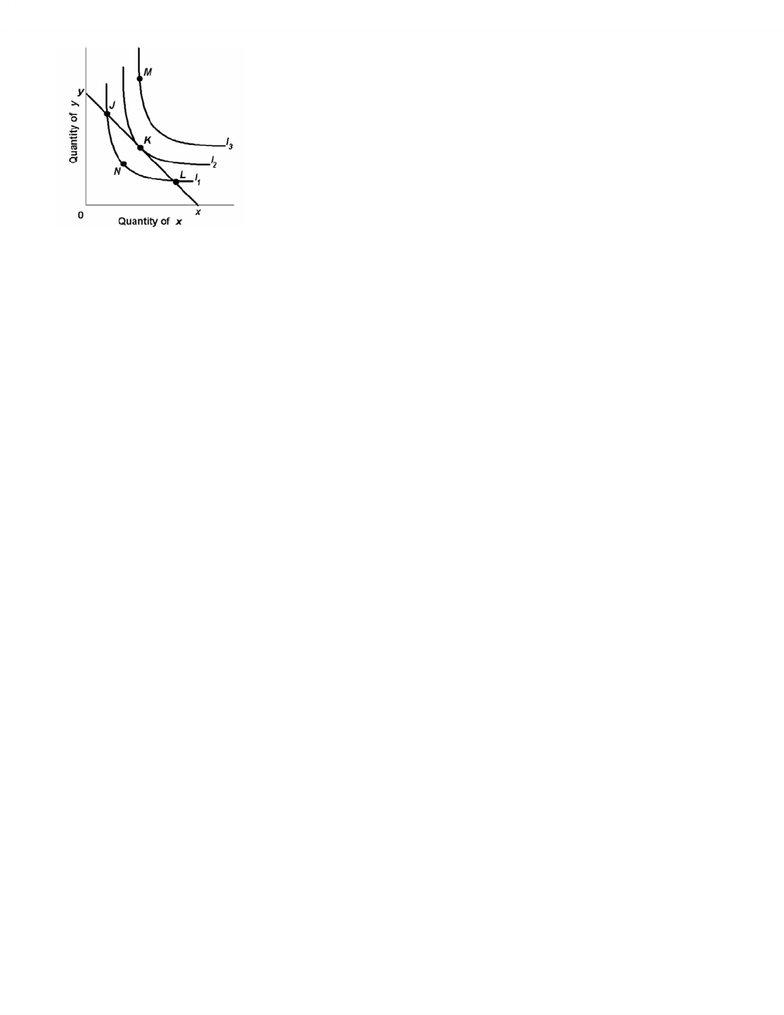# ECN 104 Lecture Notes - Normal Good, Inferior Good, Marginal Utility

374 views6 pages
School
Department
Course
ProfessorCECN 104 Name:
Seyda Yildiz ID #:
Assignment 2 (each question is worth of 3.5 points)
(due on Tuesday, May 22)
1. Which of the following statements is correct?
A. Utility and usefulness are synonymous.
B. The marginal utility derived from successive units of a product tends to be similar for all consumers.
C. Because utility is not measurable, the utility-maximizing rule provides no useful insights as to consumer
behaviour.
D. A product may yield utility, but not be functionally useful.
2. Consider Janice's consumption of wine and cheese. Suppose that the price of wine she buys is equal to the
price of cheese she buys. If Janice is a rational consumer, then we know that:
A. she buys equal quantities of each good.
B. she buys unequal quantities of each good.
C. the marginal utilities of the quantities she buys are equal.
D. the marginal utilities of the quantities she buys are unequal.
3. If a rational consumer is in equilibrium, which of the following conditions will hold true?
A. MUa = MUb = MUc = ... = MUn.
B. The marginal utility of each good purchased will be zero.
C. The marginal utility of the last dollar spent on each good purchased will be the same.
D. The total utility obtained from each good purchased will be the same.
4. If the price of normal good X rises, the income:
A. and substitution effects will both induce the consumer to buy less of X.
B. and substitution effects will both induce the consumer to buy more of X.
C. effect will induce the consumer to buy more of X and the substitution effect will induce him to buy less.
D. effect will induce the consumer to buy less of X and the substitution will induce him to buy more.
5. The price ratio of the two products is the:
A. marginal rate of substitution.
B. slope of the budget line.
C. point of tangency for equilibrium.
D. elasticity of demand for the two products.
6. Which of the following is correct?
A. Budget lines are linear and upward sloping; indifference curves are downward sloping and concave to the
origin.
B. Budget lines are linear and downward sloping; indifference curves are downward sloping and concave to the
origin.
C. Budget lines are linear and downward sloping; indifference curves are downward sloping and convex to the
origin.
D. Budget lines are downward sloping and convex to the origin; indifference curves are linear and downward
sloping.
Unlock document

This preview shows pages 1-2 of the document.
Unlock all 6 pages and 3 million more documents.7. Refer to the diagram above where xy is the relevant budget line and I1, I2, and I3 are indifference curves. The
equilibrium position for the consumer is at:
A. any point on xy.
B. point M.
C. point K.
D. point J.
8. Refer to the diagram above where xy is the relevant budget line and I1, I2, and I3 are indifference curves.
Point M:
A. is the consumer's equilibrium position.
B. is unobtainable.
C. is inferior to point N.
D. entails the highest attainable level of total utility.
9. Suppose X is a normal good. If the price of X decreases:
A. the income and substitution effects will both cause the consumer to buy less of X.
B. the income and substitution effects will both induce the consumer to buy more of X.
C. the income effect will cause the consumer to buy more of X and the substitution effect will cause the
D. the income effect will cause the consumer to buy less of X and the substitution will cause him to buy more.
10. If a product has a diminishing but positive marginal utility, then:
A. a reduction in consumption by one unit will increase total utility.
B. the product cannot be an inferior good.
C. total utility increases at a diminishing rate.
D. total utility decreases at a diminishing rate.
11. Other things equal, if the marginal utility from successive units of product Y yields to smaller and smaller
amounts of extra satisfaction, we would expect the consumer:
B. will buy less units of Y if its price decreases.
D. will not change the units of Y purchased.
12. A consumer, faced with a fixed income and constant prices for all goods consumed, can only increase total
utility if:
A. the ratios of marginal utilities to prices are not equal for all goods consumed.
B. all goods consumed have diminishing marginal utility.
C. total utility is less than marginal utility.
D. total utility is greater than marginal utility.
Unlock document

This preview shows pages 1-2 of the document.
Unlock all 6 pages and 3 million more documents.

## Document Summary

Assignment 2 (each question is worth of 3. 5 points) Suppose that the price of wine she buys is equal to the price of cheese she buys. Refer to the diagram above where xy is the relevant budget line and i1, i2, and i3 are indifference curves. The equilibrium position for the consumer is at: any point on xy, point m, point k, point j, refer to the diagram above where xy is the relevant budget line and i1, i2, and i3 are indifference curves. Point m: is the consumer"s equilibrium position, is unobtainable, is inferior to point n, entails the highest attainable level of total utility, suppose x is a normal good. The marginal utility of alpha is 40 and the marginal utility of beta is 20. A consumer is in equilibrium and is spending income in such a way that the marginal utility of product x is.

# Get access

\$10 USD/m
Billed \$120 USD annually
Homework Help
Class Notes
Textbook Notes